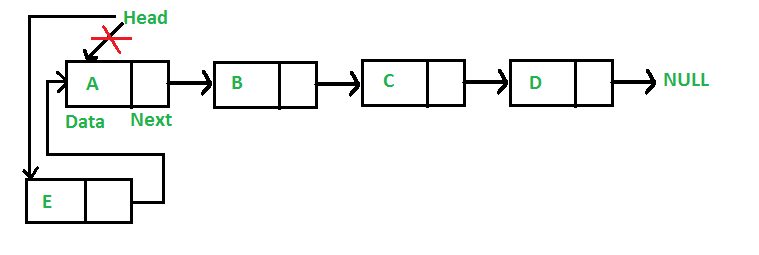Open in App
Not now

# Insert a Node at Front/Beginning of a Linked List

• Last Updated : 18 Nov, 2022

Like arrays, Linked List is a linear data structure. Unlike arrays, linked list elements are not stored at a contiguous location; the elements are linked using pointers. They include a series of connected nodes. Here, each node stores the data and the address of the next node.

Given a linked list, the task is to insert a new node at the beginning of the linked list.

Example:

List = 2->3->4->5, Insert a node with value 1 at beginning.
Output list will be: 1->2->3->4->5

Consider the following representations of the linked list.

## C++

```// A linked list node
class Node {
public:
int data;
Node* next;
};```

## C

```// A linked list node
struct Node {
int data;
struct Node* next;
};```

## Java

```// Linked List Class

/* Node Class */
class Node {
int data;
Node next;

// Constructor to create a new node
Node(int d)
{
data = d;
next = null;
}
}
}```

## Python3

```# Node class
class Node:

# Function to initialize the node object
def __init__(self, data):
self.data = data  # Assign data
self.next = None  # Initialize next as null

# Function to initialize the Linked List object
def __init__(self):
```

## C#

```/* Linked list Node*/
public class Node {
public int data;
public Node next;
public Node(int d)
{
data = d;
next = null;
}
}```

## Javascript

```<script>

/* Node Class */
class Node {

// Constructor to create a new node
constructor(d) {
this.data = d;
this.next = null;
}
}
// This code is contributed by todaysgaurav
</script>
```

Approach: The below steps should be followed to insert a new node at the front of the linked list

• Allocate a new node (say temp).
• Put the required data into temp.
• The ‘next’ pointer of the node should be pointed to the current head.
• Now make the head pointer point to temp.

See the below image for a better understanding:Insert a new node at front of Linked List

Below is the implementation of the approach:

## C++

```// Given a reference (pointer to pointer)
// to the head of a list and an int,
// inserts a new node on the front of
// the list.
{

// 1. allocate node
Node* new_node = new Node();

// 2. put in the data
new_node->data = new_data;

// 3. Make next of new node as head

// 4. Move the head to point to
// the new node
}```

## C

```/* Given a reference (pointer to pointer) to the head of a
list
and an int,  inserts a new node on the front of the list.
*/
void push(struct Node** head_ref, int new_data)
{
/* 1. allocate node */
struct Node* new_node
= (struct Node*)malloc(sizeof(struct Node));

/* 2. put in the data  */
new_node->data = new_data;

/* 3. Make next of new node as head */

/* 4. move the head to point to the new node */
}```

## Java

```/* This function is in LinkedList class. Inserts a
new Node at front of the list. This method is
defined inside LinkedList class shown above */
public void push(int new_data)
{
/* 1 & 2: Allocate the Node &
Put in the data*/
Node new_node = new Node(new_data);

/* 3. Make next of new Node as head */

/* 4. Move the head to point to new Node */
}```

## Python3

```# This function is in LinkedList class
# Function to insert a new node at the beginning
def push(self, new_data):

# 1 & 2: Allocate the Node &
# Put in the data
new_node = Node(new_data)

# 3. Make next of new Node as head

# 4. Move the head to point to new Node
```

## C#

```/* Inserts a new Node at front of the list. */
public void push(int new_data)
{
/* 1 & 2: Allocate the Node &
Put in the data*/
Node new_node = new Node(new_data);

/* 3. Make next of new Node as head */

/* 4. Move the head to point to new Node */
}```

## Javascript

```<script>

/* This function is in LinkedList class. Inserts a
new Node at front of the list. This method is
defined inside LinkedList class shown above */

function push(new_data)
{
/* 1 & 2: Allocate the Node &
Put in the data*/
var new_node = new Node(new_data);

/* 3. Make next of new Node as head */

/* 4. Move the head to point to new Node */